# Integrating electric field of rod

• ChiralSuperfields
There are several ways to proceed when trying to integrate the y-component of the electric field of a rod.

#### ChiralSuperfields

Homework Statement
Relevant Equations
For this problem,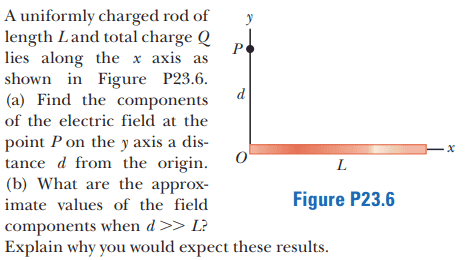However, I am trying to solve this problem using an alternative method compared with the solutions. My method is:

##\vec E = k_e \int \frac {dq} {r^2} \, dx ## ##\hat r##
##\vec E = k_e \int \frac {\lambda} {x^2 + d^2} \, dx## ## \hat r##

If I let ## \hat r = \frac {-x\hat i + d\hat j} {\sqrt {x^2 + d^2}}## then I get the same answer as the solutions.

However, how dose ## \hat r = \frac {-x\hat i + d\hat j} {\sqrt {x^2 + d^2}}##?

I see intuitively that the ##\hat r## dose point in the same direction as the ##d\vec E## for each charge segment ##dq##.

Many thanks!

Last edited:
Callumnc1 said:
##\vec E = k_e \int \frac {dq} {r^2} \, dx ## ##\hat r##
You mean ##\vec E = k_e \int \frac {dq} {r^2} \hat r##
Callumnc1 said:
dose ## \hat r = \frac {-x\hat i + d\hat j} {\sqrt {x^2 + d^2}}##?
Of course. What is the vector from (x,0) to (0,d)?
What is its magnitude?
So what is the unit vector pointing from (x,0) to (0,d)?

•ChiralSuperfields
haruspex said:
You mean ##\vec E = k_e \int \frac {dq} {r^2} \hat r##

Of course. What is the vector from (x,0) to (0,d)?
What is its magnitude?
So what is the unit vector pointing from (x,0) to (0,d)?
Thank you for your reply @haruspex ! Yes, thanks for pointing that out: I did mean ##\vec E = k_e \int \frac {dq} {r^2} \hat r##.

The vector pointing from (x,0) to (0,d) is ##\vec r = -x\hat i + d\hat j ## and its magnitude is ##\sqrt{x^2 + d^2}## so I guess we must divide the ##\vec r## but it's magnitude to find its direction to get ## \hat r = \frac {-x\hat i + d\hat j} {\sqrt {x^2 + d^2}}##

Then we just translate the unit vector ##\hat r## to the right for a charge element ##dq## as shown below: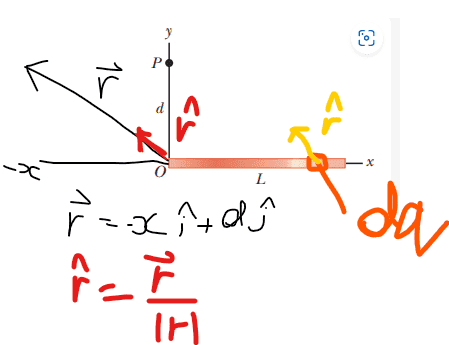Many thanks!

#### Attachments

Callumnc1 said:
Thank you for your reply @haruspex ! Yes, thanks for pointing that out: I did mean ##\vec E = k_e \int \frac {dq} {r^2} \hat r##.

The vector pointing from (x,0) to (0,d) is ##\vec r = -x\hat i + d\hat j ## and its magnitude is ##\sqrt{x^2 + d^2}## so I guess we must divide the ##\vec r## but it's magnitude to find its direction to get ## \hat r = \frac {-x\hat i + d\hat j} {\sqrt {x^2 + d^2}}##

Then we just translate the unit vector ##\hat r## to the right for a charge element ##dq## as shown below:

View attachment 320665

Many thanks!
Yes.

•ChiralSuperfields
haruspex said:
Yes.
Ok thank you for your help @haruspex !

So my question was about integrating the y-component of the electric field of the rod. I am not sure how to integrate ## E_y = k_ed\lambda\int \frac {dx} {(x^2 + d^2)^{3/2}} \ ##

Many thanks!

Callumnc1 said:

So my question was about integrating the y-component of the electric field of the rod. I am not sure how to integrate ## E_y = k_ed\lambda\int \frac {dx} {(x^2 + d^2)^{3/2}} \ ##

Many thanks!
There are several ways to proceed.
You can try using a trig substitution to get rid of the square root.
Another way is to turn x.dx into d(x2)/2.
Another is to guess that ##(x^2 + d^2)^{1/2}## may appear in the answer, and differentiate that to see what you get.

Sorry… just realised you are asking about ## E_y = k_ed\lambda\int \frac {dx} {(x^2 + d^2)^{3/2}} \ ##, not ## E_y = k_ed\lambda\int \frac {xdx} {(x^2 + d^2)^{3/2}} \ ##, so the last two won't work.

Bear in mind that (1) an answer in the form of an integral may be acceptable for part a), and (2) part b) asks for an approximation, which suggests that one or both of the integrals cannot be solved exactly.

•MatinSAR and ChiralSuperfields
haruspex said:
There are several ways to proceed.
You can try using a trig substitution to get rid of the square root.
Another way is to turn x.dx into d(x2)/2.
Another is to guess that ##(x^2 + d^2)^{1/2}## may appear in the answer, and differentiate that to see what you get.

Sorry… just realised you are asking about ## E_y = k_ed\lambda\int \frac {dx} {(x^2 + d^2)^{3/2}} \ ##, not ## E_y = k_ed\lambda\int \frac {xdx} {(x^2 + d^2)^{3/2}} \ ##, so the last two won't work.

Bear in mind that (1) an answer in the form of an integral may be acceptable for part a), and (2) part b) asks for an approximation, which suggests that one or both of the integrals cannot be solved exactly.

I can solve the first integral for the x-component of the electric field, however, I have not done trig substitution yet.

All the solutions say for the integration of the y-component of the electric field is: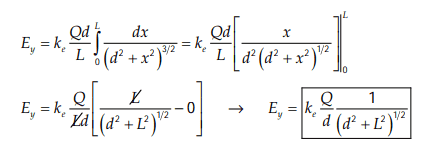Thank you!

Callumnc1 said:

I can solve the first integral for the x-component of the electric field, however, I have not done trig substitution yet.

All the solutions say for the integration of the y-component of the electric field is:
View attachment 320704
Thank you!
You can check that easily enough by differentiating the result.
For trig substitution, what two trig functions do you know that satisfy ##g^2=f^2+1##?

•MatinSAR and ChiralSuperfields
haruspex said:
You can check that easily enough by differentiating the result.
For trig substitution, what two trig functions do you know that satisfy ##g^2=f^2+1##?

There are two trig identities I'm aware of that satisfy ##g^2=f^2+1##:

1. ## \sec^2\theta = \tan^2\theta + 1##
2. ## \csc^2\theta = \cot^2\theta +1 ##

Which two trig functions were you looking for?

Thank you!

Callumnc1 said:

There are two trig identities I'm aware of that satisfy ##g^2=f^2+1##:

1. ## \sec^2\theta = \tan^2\theta + 1##
2. ## \csc^2\theta = \cot^2\theta +1 ##

Which two trig functions were you looking for?

Thank you!
The first will do. Can you see how to use it to get rid of the square root in the denominator?

•MatinSAR and ChiralSuperfields
haruspex said:
The first will do. Can you see how to use it to get rid of the square root in the denominator?
Thanks for your reply @haruspex ! I think so - I'll give it I try!

haruspex said:
The first will do. Can you see how to use it to get rid of the square root in the denominator?
So far I have got:

## (\sec^2\theta)^{1/3} = (\tan^2\theta + 1)^{1/3}##
## (\sec\theta)^{2/3} = (\tan^2\theta + 1)^{1/3}##
## \sec\theta = d^2 + x^2 ## from comparison
## x = (\sec\theta - d^2)^{1/2} ##
## dx = \frac {\sec\theta\tan\theta} {2\sqrt{\sec\theta - d^2}} d\theta ##

Callumnc1 said:
## \sec\theta = d^2 + x^2 ##
No, you can't do that. It's dimensionally invalid.
Besides, you want ##d^2 + x^2 ## to be something squared so that you can take its square root.
Try ##x=d\times## a trig function.

•MatinSAR and ChiralSuperfields
Callumnc1 said:
So far I have got:

## (\sec^2\theta)^{1/3} = (\tan^2\theta + 1)^{1/3}##
## (\sec\theta)^{2/3} = (\tan^2\theta + 1)^{1/3}##
## \sec\theta = d^2 + x^2 ## from comparison
## x = (\sec\theta - d^2)^{1/2} ##
## dx = \frac {\sec\theta\tan\theta} {2\sqrt{\sec\theta - d^2}} d\theta ##
Thanks for using LaTeX !

You have some things mixed up. Also, using "d" (lower case D) as a symbol in this problem is a bad idea, but that's what was given to you., so let's go with it.

Actually, you want to let ##\displaystyle \tan \theta = \dfrac{x}{(d)} ## , so that ##\displaystyle (d) \tan \theta = x ##

That gives you ##\displaystyle ((d)^2 + x^2)^{(3/2)}=((d)^2 + (d)^2 \tan^2 \theta)^{(3/2)}=(d)^3(1 +\tan^2 \theta)^{(3/2)}## for your denominator.

The change of variable gives you ##\displaystyle dx = (d)\sec^2 \theta \, d\theta## .

Last edited:
•ChiralSuperfields
SammyS said:
Thanks for using LaTeX !

You have some things mixed up. Also, using "d" (lower case D) as a symbol in this problem is a bad idea, but that's what was given to you., so let's go with it.

Actually, you want to let ##\displaystyle \tan \theta = \dfrac{x}{(d)} ## , so that ##\displaystyle (d) \tan \theta = x ##

That gives you ##\displaystyle ((d)^2 + x^2)^{(3/2)}=((d)^2 + (d)^2 \tan^2 \theta)^{(3/2)}=(d)^3(1 +\tan^2 \theta)^{(3/2)}## for your denominator.

The change of variable gives you ##\displaystyle dx = (d)\sec^2 \theta \, d\theta## .
Sorry for my very late reply - Thank you @haruspex and @SammyS for your replies! I'll see if I can try that out tomorrow, sorry I'm a bit busy at the moment.
haruspex said:
No, you can't do that. It's dimensionally invalid.
Besides, you want ##d^2 + x^2 ## to be something squared so that you can take its square root.
Try ##x=d\times## a trig function.
Also, was it it dimensionally invalid? Is it because there is meters (which dimension is length) on one side and no dimensions on the other side?

Thank you!

Callumnc1 said:
Is it because there is meters (which dimension is length) on one side and no dimensions on the other side?
Yes.

•ChiralSuperfields and MatinSAR
haruspex said:
Yes.
Thank you @haruspex ! I don't see how you get a conflicting result when you change the units, to cm say. Could you please explain?

Thank you!

Last edited:
SammyS said:
Thanks for using LaTeX !

You have some things mixed up. Also, using "d" (lower case D) as a symbol in this problem is a bad idea, but that's what was given to you., so let's go with it.

Actually, you want to let ##\displaystyle \tan \theta = \dfrac{x}{(d)} ## , so that ##\displaystyle (d) \tan \theta = x ##

That gives you ##\displaystyle ((d)^2 + x^2)^{(3/2)}=((d)^2 + (d)^2 \tan^2 \theta)^{(3/2)}=(d)^3(1 +\tan^2 \theta)^{(3/2)}## for your denominator.

The change of variable gives you ##\displaystyle dx = (d)\sec^2 \theta \, d\theta## .
No worries @SammyS ! So solving using trig substitution:

## E_y = k_ed\lambda\int \frac {dx} {(x^2 + d^2)^{3/2}} \ ##
## E_y = k_ed\lambda\int \frac {(d)\sec^2 \theta \, d\theta} {(d)^3(1 +\tan^2 \theta)^{(3/2)}} \ ##
## E_y = k_ed\lambda\int \frac {\sec^2 \theta \, \theta} {(d)^2(1 +\tan^2 \theta)^{(3/2)}} \ ##
## E_y = k_ed\lambda\int \frac {1 +\tan^2 \theta} {(d)^2(1 +\tan^2 \theta)^{(3/2)}} \ ##
## E_y = k_ed\lambda\int \frac {1} {(d)^2(1 +\tan^2 \theta)^{(1/2)}} \ ##

I'm not sure how to finish from here. Have I done it correctly so far?

EDITED II: I also found this on an integral table: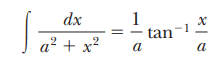However, I think this only holds for when the power of the brackets for the bottom fraction is equal to 1. Am I correct?

Many thanks!

Last edited:
Callumnc1 said:
Thank you @haruspex ! I don't see how you get a conflicting result when you change the units, to cm say. Could you please explain?

Thank you!
Suppose d and x are 3cm and 4cm. Your expression in post #13 gives ##\sec(\theta)=25##. But putting them in m gives ##\sec(\theta)=.00025##.

•ChiralSuperfields
Callumnc1 said:
how to finish from here.
Instead of converting ##\sec^2## to ##1+\tan^2## in the numerator, try the reverse conversion in the denominator.
Btw, you have dropped ##d\theta##.

•ChiralSuperfields
Callumnc1 said:
No worries @SammyS ! So solving using trig substitution:

## E_y = k_ed\lambda\int \frac {dx} {(x^2 + d^2)^{3/2}} \ ##
## E_y = k_ed\lambda\int \frac {(d)\sec^2 \theta \, d\theta} {(d)^3(1 +\tan^2 \theta)^{(3/2)}} \ ##
## E_y = k_ed\lambda\int \frac {\sec^2 \theta \, \theta} {(d)^2(1 +\tan^2 \theta)^{(3/2)}} \ ##
## E_y = k_ed\lambda\int \frac {1 +\tan^2 \theta} {(d)^2(1 +\tan^2 \theta)^{(3/2)}} \ ##
## E_y = k_ed\lambda\int \frac {1} {(d)^2(1 +\tan^2 \theta)^{(1/2)}} \ ##

I'm not sure how to finish from here. Have I done it correctly so far?

EDITED: I also found this on an integral table:
View attachment 321000
However, I think this only holds for when the power of the brackets for the bottom fraction is equal to 1.
Change ##\displaystyle 1+ \tan^2 \theta## to ##\displaystyle \sec^2 \theta## rather than the other way around. Pull the ##\dfrac 1 {(d)^2}## out of the integral. Also, you have somehow dropped the ##d\theta## from the integral. (maybe a typo)

•ChiralSuperfields
haruspex said:
Suppose d and x are 3cm and 4cm. Your expression in post #13 gives ##\sec(\theta)=25##. But putting them in m gives ##\sec(\theta)=.00025##.
Thank you @haruspex for the example - I see now!

Thank you for your replies @SammyS and @haruspex ! Sorry it dose look like I dropped the ##d\theta##

## E_y = k_ed\lambda\int \frac {\sec^2 \theta \, d\theta} {(d)^2(sec^2 \theta)^{(3/2)}} \ ##
## E_y = k_ed^{-1}\lambda\int \frac {\sec^2 \theta \, d\theta} {(sec \theta)^3} \ ##
## E_y = k_ed^{-1}\lambda\int \frac {1} {(sec \theta)} \, d\theta##
## E_y = k_ed^{-1}\lambda\int cos\theta \, d\theta##
## E_y = k_ed^{-1}\lambda sin\theta ##

Is it so far correct? I should probably add some limits of integration e.g ## \theta_1 = 0 ## and ## \theta_2 = 90 ##

Last edited:
Callumnc1 said:
Thank you for your replies @SammyS and @haruspex ! Sorry it dose look like I dropped the ##d\theta##

## E_y = k_ed\lambda\int \frac {\sec^2 \theta \, d\theta} {(d)^2(sec^2 \theta)^{(3/2)}} \ ##
## E_y = k_ed^{-1}\lambda\int \frac {\sec^2 \theta \, d\theta} {(\sec \theta)^3} \ ##
## E_y = k_ed^{-1}\lambda\int \frac {1} {(\sec \theta)} \, d\theta##
## E_y = k_ed^{-1}\lambda\int \cos\theta \, d\theta##
## E_y = k_ed^{-1}\lambda \sin\theta ##

Is it so far correct? I should probably add some limits of integration e.g ## \theta_1 = 0 ## and ## \theta_2 = 90 ##
Not the correct limits of integration.
You can write ##\sin \theta## in terms of ##x## and integrate from ##0## to ##L##, the original limits of integration.
Or you can find the limits of integration you need for ##\theta##.

•ChiralSuperfields
SammyS said:
Not the correct limits of integration.
You can write ##\sin \theta## in terms of ##x## and integrate from ##0## to ##L##, the original limits of integration.
Or you can find the limits of integration you need for ##\theta##.
Thank you for you reply @SammyS !

## E_y = k_ed^{-1}\lambda\int_0^{\theta_L} cos\theta \, d\theta##
## E_y = k_ed^{-1}\lambda sin\theta_L ##

Where ##\theta_L## is the angle the position vector makes with in the ##-\hat j## direction to the end of the rod.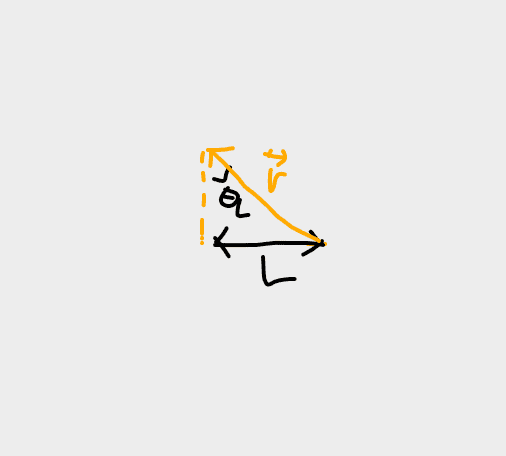## E_y = k_e\lambda \frac {L} {d(L^2 + d^2)^{1/2}} ##
## E_y = \frac {k_eQ} {d(L^2 + d^2)^{1/2}} ##

Which is the same answer as the solutions! :)

Last edited:
•SammyS